# How to create matrix questions

This document’s purpose is to explain how to create matrix questions.

## Adding questions to a question pool

1. To add questions to a question pool, navigate to “Tests/Question pools.”2. To add questions to a new question pool, click the “New” button.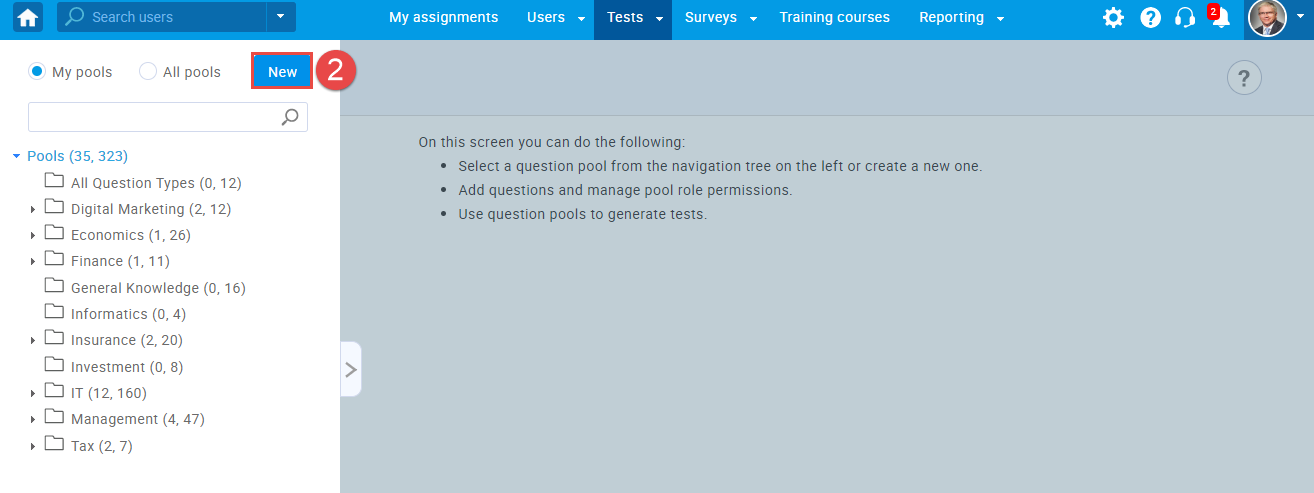3. In the popup window, add a pool name and save it.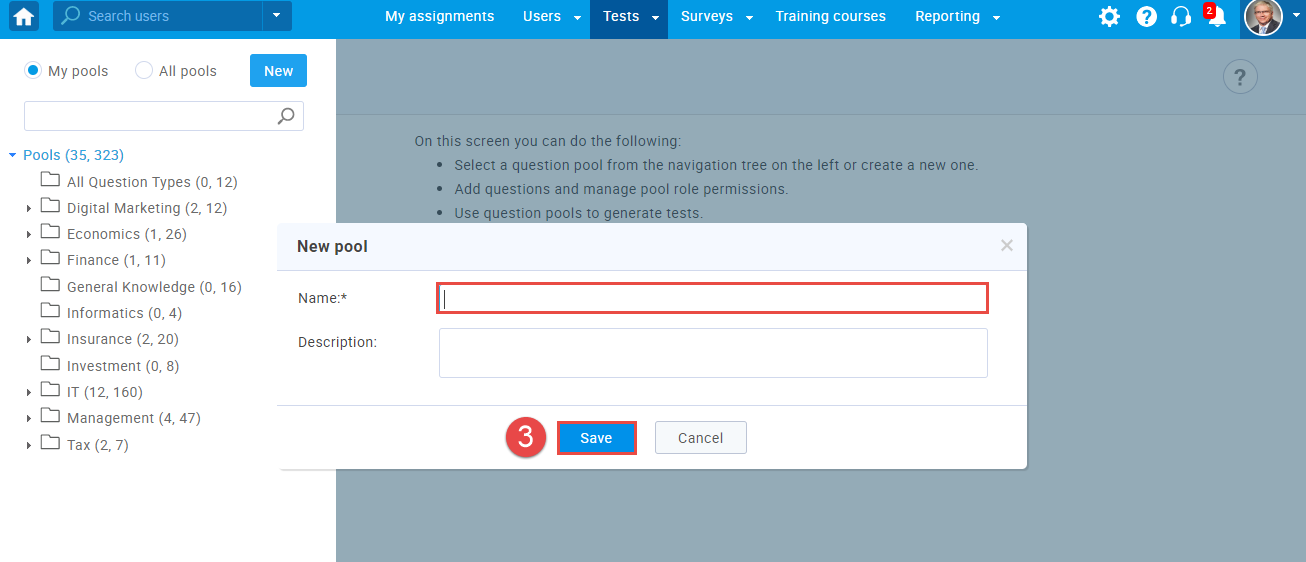4. To add questions to an existing question pool, select the pool name from the list of pools.## Matrix questions

1. To add a matrix question to a newly created or an existing question pool, select the “Questions” tab.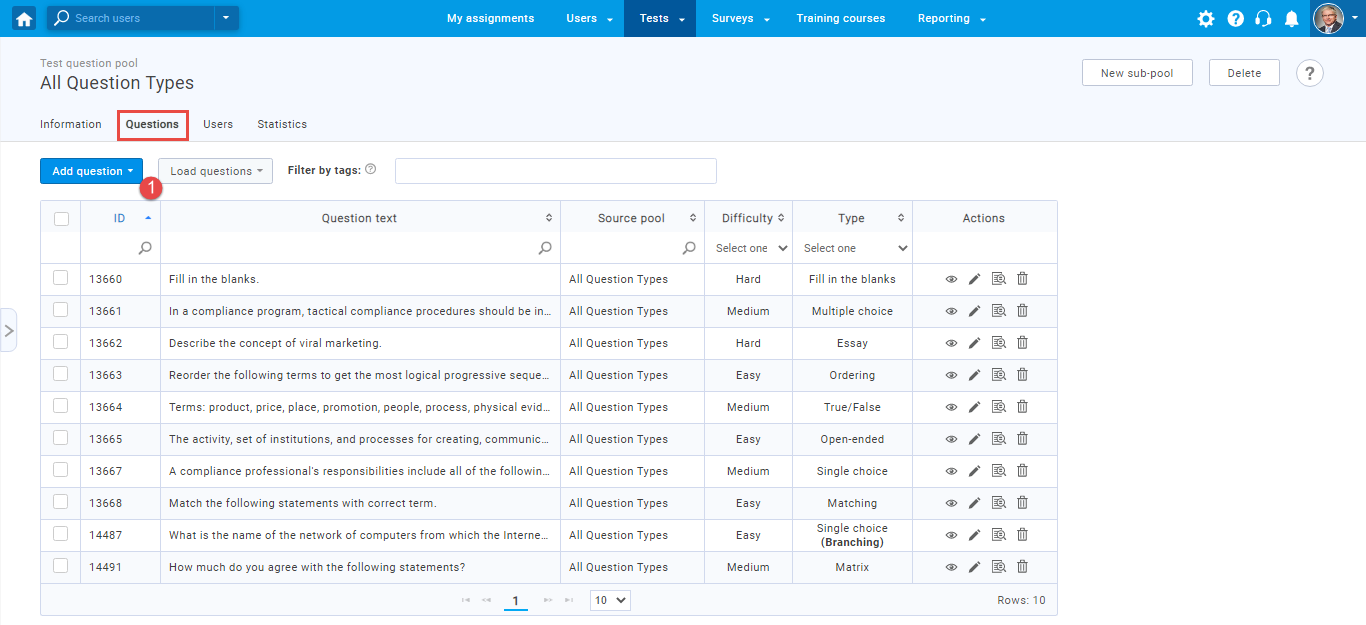2. Click on the “Add question” button and select a matrix question.3. Configure the question settings (difficulty, duration, scoring method, tags, points, penalty, frequency).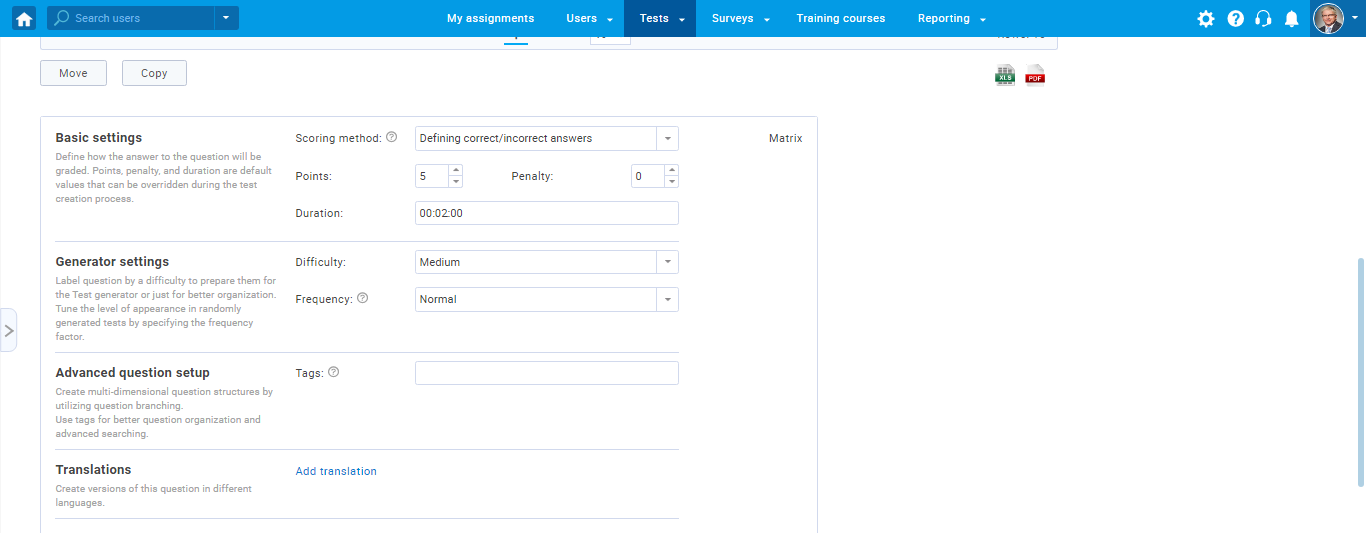• Scoring method – User can choose between three scoring methods:
• Defining correct/incorrect answers – Candidate has to select all of the correct answers to achieve a point
• By answer weight – Each answer has its weight. For every answer, a user picks he will be awarded points accordingly (depending on the weight)
• Manual grading – Candidate earns points after the test manager grades the question
4. Adding question introduction – Here you can enter a question introduction.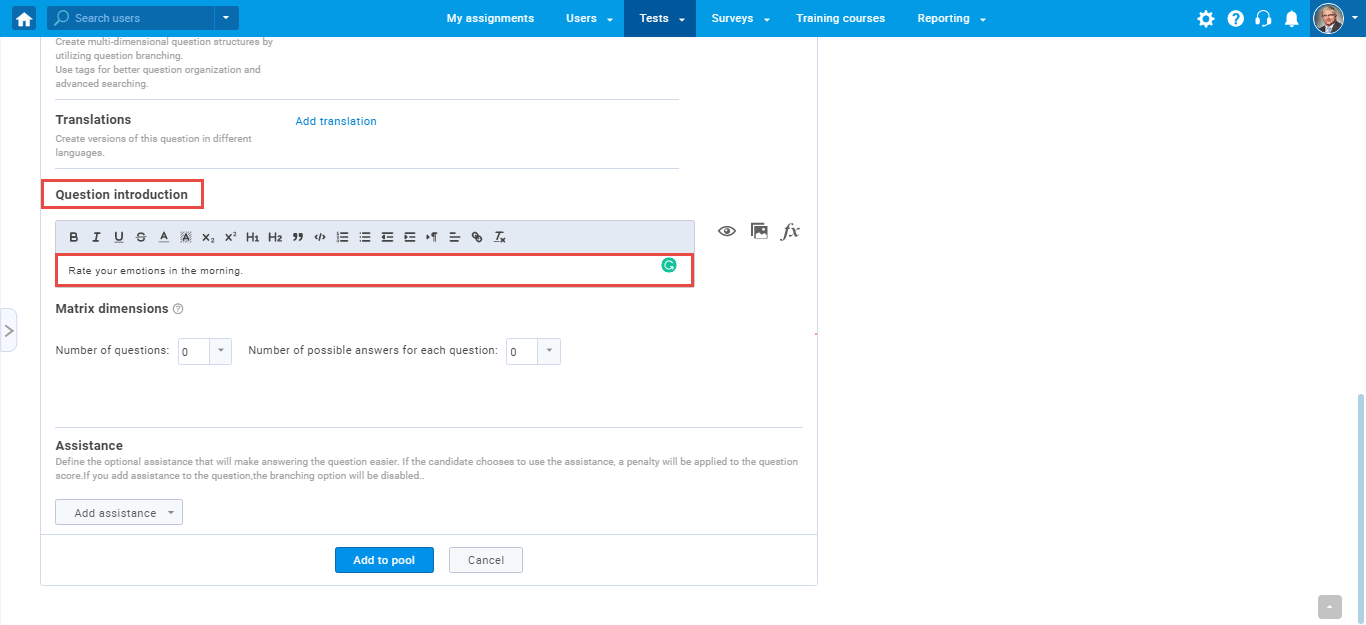5. Adding questions – Select the number of questions that will be presented to the user and write the questions in the box.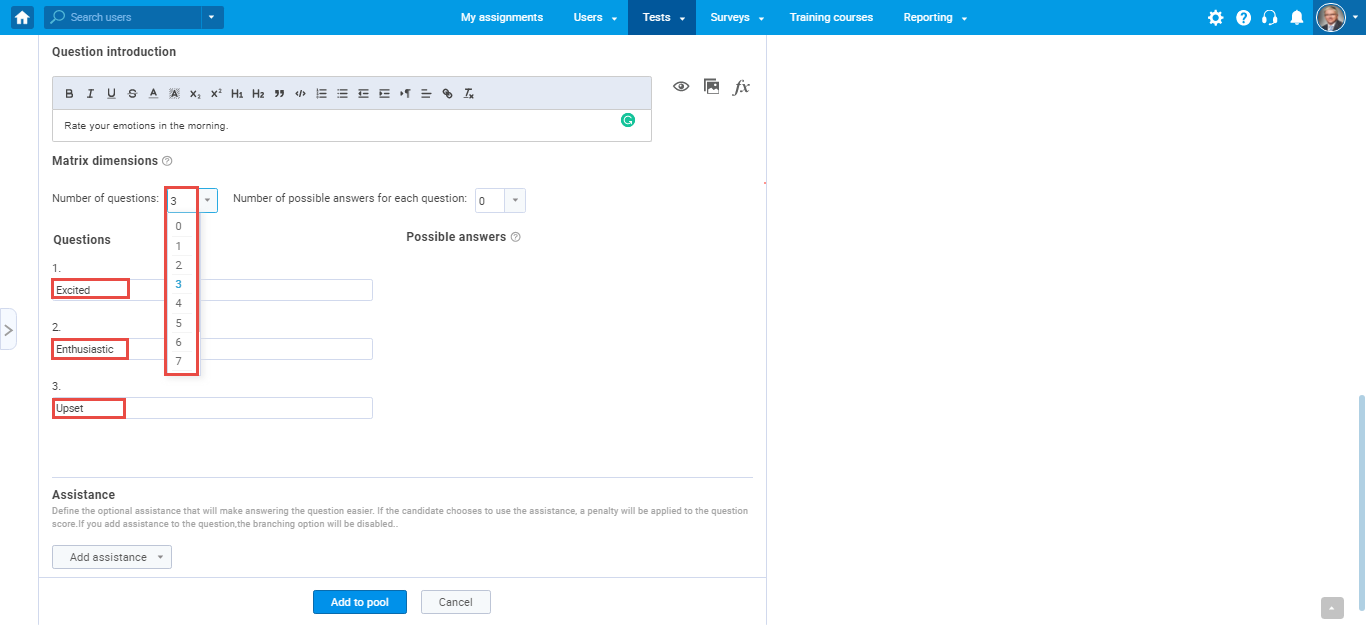6. Adding possible answers – Choose the number of answers a user will be able to choose.## Matrix question from a students perspective

The test taker will need to choose from the following statements and indicate the extent to which he agrees with each of them).